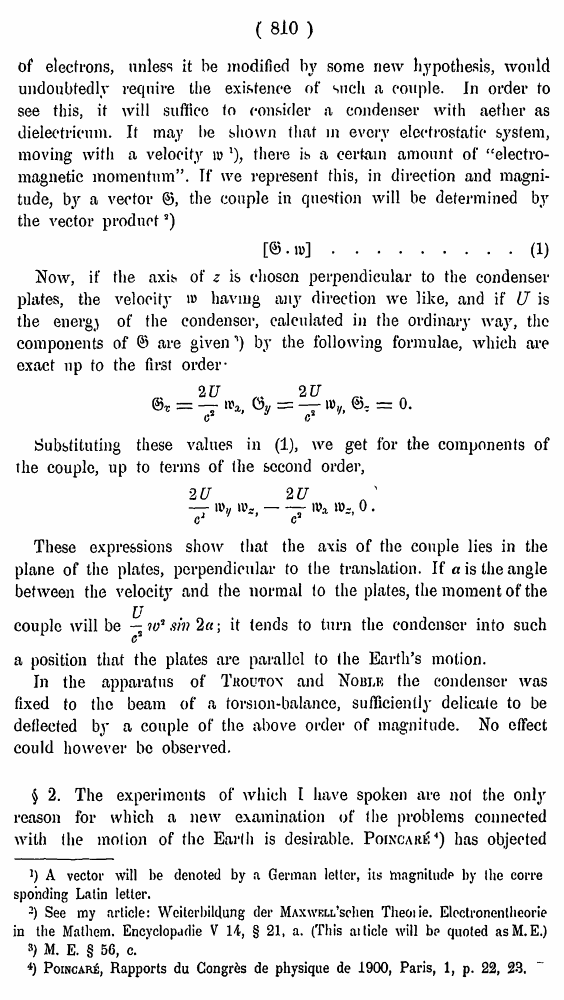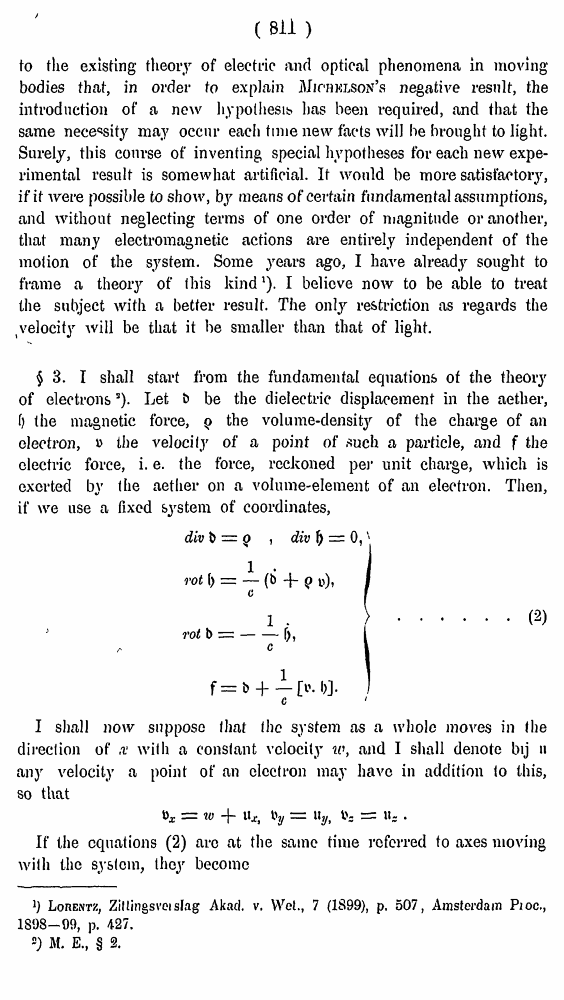# H. A. Lorentz. Electromagnetic phenomena in a system moving with any velocity smaller than that of light. // Proceedings Royal Acad., Amsterdam. Vol. VI., 1904

В начало   Другие форматы   <<<     Страница 810   >>>809  810 811  812  813  814  815  816  817  818  819  820  821  822  823  824  825  826  827  828  829  830  831

( 810 )

of electrons, unless it be modified by some new hypothesis, would undoubtedly require the existence of such a couple. In order to see this, it will suffice to consider a condenser with aether as dielectricum. It may be shown that in every electrostatic system, moving with a velocity w x), there is a certain amount of “electromagnetic momentum”. If we represent this, in direction and magnitude, by a vector ©, the couple in question will be determined by the vector product3)

### .........(1)

Now, if the axis of z is chosen perpendicular to the condenser plates, the velocity u> having any direction we like, and if U is the energy of the condenser, calculated in the ordinary way, the components of © are given') by the following formulae, which are exact up to the first order-

Substituting these values in (1), we get for the components of the couple, up to terms of the second order,

These expressions show that the axis of the couple lies in the plane of the plates, perpendicular to the translation. If a is the angle between the velocity and the normal to the plates, the moment of the

couplc will be; it tends to turn the condenser into such

a position that the plates are parallel to (he Earth’s motion.

In the apparatus of Trouton and Noble the condenser was fixed to the beam of a torsion-balance, sufficiently delicate to be deflected by a couple of the above order of magnitude. No effect could however be observed.

§ 2. The experiments of which I have spoken are not the only reason for which a new examination of the problems connected with the motion of the Earlli is desirable. Poincare4) has objected

]) A vector will be denoted by a German letter, its hiagnitudp by the corre spo'nding Latin letter.

2) See my article: Weiterbildung der Maxwell’schen Theorie. Electronentlieorie in the Mathem. Encyclopadie V 14, § 21, a. (This ai ticle will bp quoted asM.E.)

s) M. E. § 56, c. ’

4) Poincar£, Rapports du Congres de physique de 1900, Paris, 1, p. 22, 23.

###### (sn >

to the existing theory of electric and optical phenomena in moving bodies that, in order to explain Michelson’s negative result, the introduction of a new hypothesis has been required, and that the same necessity may occur each time new facts will be brought to light. Surely, this course of inventing special hypotheses for each new experimental result is somewhat artificial. It would be more satisfactory, if if were possible to show, by means of certain fundamental assumptions, and without neglecting terms of one order of magnitude or another, that many electromagnetic actions are entirely independent of the motion of the system. Some years ago, I have already sought to frame a theory of this kind1). I believe now to be able to treat the subject with a better result. The only restriction as regards the velocity will be that it be smaller than that of light.

\$3. I shall start from the fundamental equations of the theory of electrons3). Let & be the dielectric displacement in the aether, f) the magnetic force, q the volume-density of the charge of an electron, » the velocity of a point of such a particle, and f the electric force, i. e. the force, reckoned per unit charge, which is exerted bv the aether on a volume-element of an electron. Then, if we use a fixed system of coordinates,

I shall now suppose I hat the system as a whole moves in the direction of x with a constant velocity to, and I shall denote bij h any velocity a point of an electron may have in addition to this, so that

If the equations (2) arc at the same time referred to axes moving with the system, they become

», = «> + u* i>y = %, .

]) Lorentz, Zil lings vet slag Akad. v. Wet., 7 (1899), p. 507, Amsterdam Proc., 1898-09, p. 427.

2) M. E., § 2.